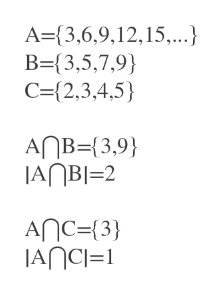Define the following sets.A = {x ∈ Z: x is a multiple of 3}B = {3, 5, 7, 9}C = {2, 3, 4, 5}Indicate whether each statement is true or false.(a)|B| = |C| (b)|A ∩ B| = |A ∩ C| (c)A ∩ C ⊆ A ∩ B (d)C - B ⊆ A (e)B ∪ C = {3, 5} (f)2 ∈ A ∪ C (g){2, 3} ∈ C (h){3} ∈ P(C) (i)A ∩ B ∩ C = ∅ (j)∅ ∈ A

Question

Define the following sets.
A = {x ∈ Z: x is a multiple of 3}
B = {3, 5, 7, 9}
C = {2, 3, 4, 5}
Indicate whether each statement is true or false.
(a)
|B| = |C|
(b)
|A ∩ B| = |A ∩ C|
(c)
A ∩ C ⊆ A ∩ B
(d)
C - B ⊆ A
(e)
B ∪ C = {3, 5}
(f)
2 ∈ A ∪ C
(g)
{2, 3} ∈ C
(h)
{3} ∈ P(C)
(i)
A ∩ B ∩ C = ∅
(j)
∅ ∈ A

Step 1

Since we only answer up to 3 sub-parts, we’ll answer the first 3. Please resubmit the question and specify the other subparts (up to 3) you’d like answered.

A = {x ∈ Z: x is a multiple of 3}={3,6.9,12,15,.....}

B = {3, 5, 7, 9}
C = {2, 3, 4, 5}

Step 2

a. B = {3, 5, 7, 9}
C = {2, 3, 4, 5}

|B|= number of elements in B= 4

|C|=number of elements in C=4

So, |B|=|C| is true.

Step 3

|A ∩ B|= 2 and |A ∩ C|=1

So b is not ...help_outlineImage TranscriptioncloseA-(3,6,9,12,15,..} B={3,5,7,9) C=(2,3,4,5 AnB {3,9 JANBI-2 AnC={3} JANCI=1 fullscreen

Want to see the full answer?

See Solution

Want to see this answer and more?

Our solutions are written by experts, many with advanced degrees, and available 24/7

See Solution
Tagged in

Algebra